Sie sind hier: ICP » R. Hilfer » Publikationen

# 3 Fractional dynamics (FD) and excess wings

[123.6.1] The theory of fractional dynamics yields a three parameter function, that allows to fit both, the asymmetric peak and the excess wing with a single stretching exponent [17, 16, 23]. [123.6.2] The three parameter function is denoted as “fractional dynamics” (FD) relaxation in figure 2. [123.6.3] Its functional form reads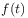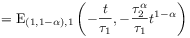(8a)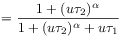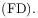(8b)

where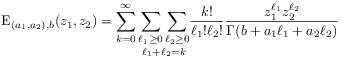(9)

with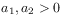and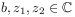is the binomial Mittag-Leffler function . [123.6.4] The function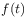from eq. (8) solves the fractional differential equation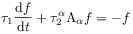(10)

with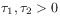,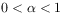, and inital value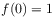. [123.6.5] In eq. (10) the operator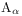is the infinitesimal generator of fractional time evolutions of index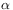[7, 11, 10, 9, 15, 23]. [123.6.6] It can be written as a fractional time derivative of orderin the form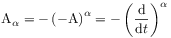(11)

where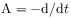is the infinitesimal generator of translations. [123.6.7] For a mathematical definition ofsee . [123.6.8] Note, that the solution (8) of eq. (10) holds also for generalized Riemann-Liouville operators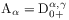of orderand type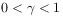as shown in [17, 16]. [123.6.9] The (right-/left-sided) generalized Riemann-Liouville fractional derivative of orderand type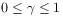with respect to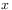was introduced in definition 3.3 in [15, p.113] by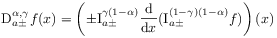(12)

where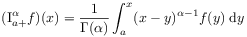(13)

for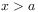, denotes the right-sided Riemann-Liouville fractional integral of order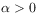, and the left sided integral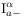is defined analogously [15, 13].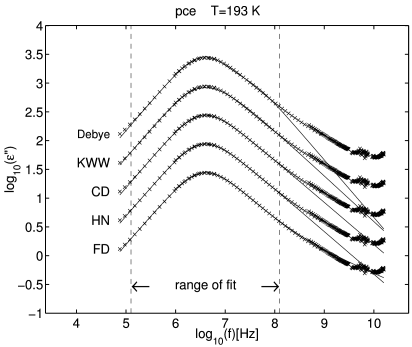Figure 2: Five different fits to the imaginary part Im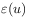(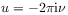) of the complex dielectric function of propylene carbonate at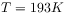as a function of frequency. Experimental data represented by crosses are taken from Ref. . The range over which the data were fitted is indicated by dashed vertical lines in the figure. For clarity the data were displaced vertically by half a decade each. The original location of the data corresponds to the curve labelled FD.